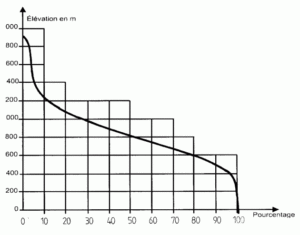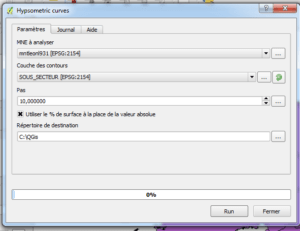# QGis 2.8 Wien: calculation of Hypsometric Curves

The new version of QGis is available.
Among the novelties announced in this version, the calculation of the curves hypsometric is included.

Here is an article to discuss how this new algorithm works.

A little reminder about the hypsometric curves

Given that most meteorological and hydrological factors are depending on altitude, it is interesting to study the hypsogram or frequency histogram of elevations of the watershed for each interval of altitude.

The histogram is a graph on which the values of the altitudes are spread in intervals on the x – axis; and in the y-axis, the surface, in % of the total area between two successive altitudes .

The hypsometric curve is a curve that can be built on the previous graph where the ordinates represent the catchment area which is located above the coast altitude defined in the abscissaThe histogram and the hypsometric curve reflect the distribution of altitudes within the watershed and allow determining the following characteristic altitudes:
* average altitude: it’s the average abscissa of the hypsometric curve.
Average altitude = ( alt.max + alt.min ) / 2, and also, following the weighting method
* Most frequent elevation: it is the maximum of the frequency of curve altimetry or, also, the altitude of the largest individual area between two successive contours.
* ½ frequency altitude: it is altitude corresponding to the ordinate point 50% of the hypsometric curve.

Recent research have shown the general aspect of the curves according to the erosion state of the watershed:-for the most recent basins (3), the area is weak if compared with the initial altitude change l, which is characteristic of steep basins;
-the old basins (1) show the opposite ; that is to say, a gentle plain near a watercourse where the altitude varies very little in spite of being a large area;
-the third case (2), is approaching to the so-called “mature” state, at least according to this degree erosion classification associated with the age of the basin .

The calculation of the hypsometric curve with QGis 2.8

We find the command in the treatment menu -> Toolbox to display the treatments window and, in the text box “Geoprocessing QGis   -> Raster tools -> Hypsometric curvesTo execute the command two layers are needed:

• a DTM (raster)
• a watershed layer (polygons)The path is defined in meters for the surfaces calculation: a step of 10 meters is chosen.

The box to check determines if the result that will be recorded in the output table is the class surface elevation or the percentage relative to the total area of the watershed.

Finally, the directory is indicated where the output will be saved. For each entity of the vector layer input , a CSV file with surface and altitude values will be created .

The name of the file consists of the prefix histogram _ followed by the name of the layer and the ID of the entity.To build the curve, just open the files created with Excel or OpenOffice Calc.

Open the file
Select the Surface and Elevation data set
Click Insert-> Graphics -> Point Cloud with gentle curves
To obtain the classical level diagram the values of ordinates have to be reversed: Click the X-axis and select “Values in reverse order”.### 6 Responses to QGis 2.8 Wien: calculation of Hypsometric Curves

1.Gabriela says:

Hello: Thank you for the comprehensive explanation. Which format should the DEM Raster be for QGIS environment?

2.G says:

Thanks for the quick answer. I was thinking of using a geotiff format and I did not know if it can support any kind of format.

•Atilio Francois says:

Yes, it does.

3.Jeevan says:

I have tried, but what I am getting an inverse curve, like max elevation is max %, is it correct ? plz let me know

•Atilio Francois says:

Sorry, I don’t have an English version of Excel. In order not to have the inverted curve, check the “Values in inverted order” (translation not guaranteed) box in the “X-axis format” panel